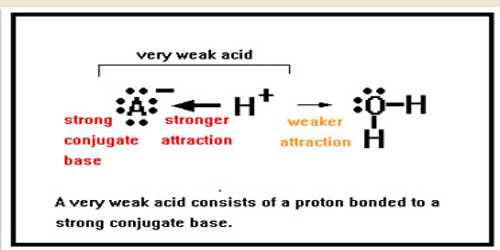# pH of Solutions of Very Weak Bases

pH of solutions of very weak bases

Unlike strong acids/bases, weak acids and weak bases do not completely dissociate (separate into ions) at equilibrium in water, so calculating the pH of these solutions requires consideration of a unique ionization constant and equilibrium concentrations. The same basic method can be used to determine the pH of aqueous solutions of many different weak acids and bases.

A treatment similar to the one used for weak acids may be given for the dissociation of weak bases. For a weak base B in aqueous solution the following equilibrium exists:

B (aq) + H2O (l) ↔ BH+ (aq) + OH (aq)The equilibrium constant Kb called the base dissociation constant, is given by;

Kb = [BH+] x [OH] / [B]

Water being the solvent its concentration is taken as constant. As the base is very weak, [B] does not change very much and may be considered constant for all practical purposes. Since, [BH+] = [OH] equation (1) may be written as-

[OH]2 = Kb [B]

so, [OH] = √( Kb [B])

Weak acids and bases are only incompletely ionized in their solutions, whereas strong acids and bases are totally ionized when dissolving in water.

The ionization of weak acids and bases is a chemical equilibrium occurrence. The equilibrium principles are necessary for the understanding of equilibria of weak acids and weak bases.